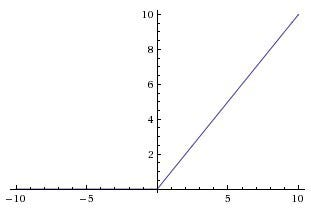# Activation Function- ReLU

Source: Deep Learning on Medium# Activation Function- ReLU

This article discusses one of the most prominent activation used in Neural Networks.

# Activation function

The main role of the activation function is to decide whether neural networks should be activated or not. This is inspired by biological neural networks. The choice of the different activation function is dependent on the architecture of the network and also on results one obtain using them.

An activation function can be Linear and Non-Linear, but a network with linear function can only learn linear problems since summing all the layers in the network will give another linear function. whereas Non-Linearity allows the network to learn more complex problems.

Some of the desirable properties of the activation function are mentioned below.

## Properties of the Activation Function

• Non-Linearity: The main purpose of the activation function is to introduce Non-linearity in the network such that the network would be capable of learning more complex patterns.
• Continuous differentiable: Activation function should be continuously differentiable with respect to the weights for the gradient-based optimization methods.
• Monotonic: Activation function helps the neural network to converge easier into a more precise model.

# What is ReLU?

ReLU function is one of the most widely used hidden layer activation function and it is non-linear, monotonic and also continuously differentiable almost everywhere (except at 0, the left derivative at z = 0 is 0 and the right derivative is 1).